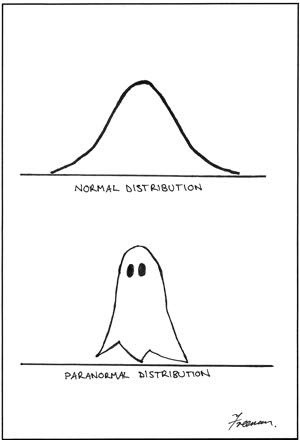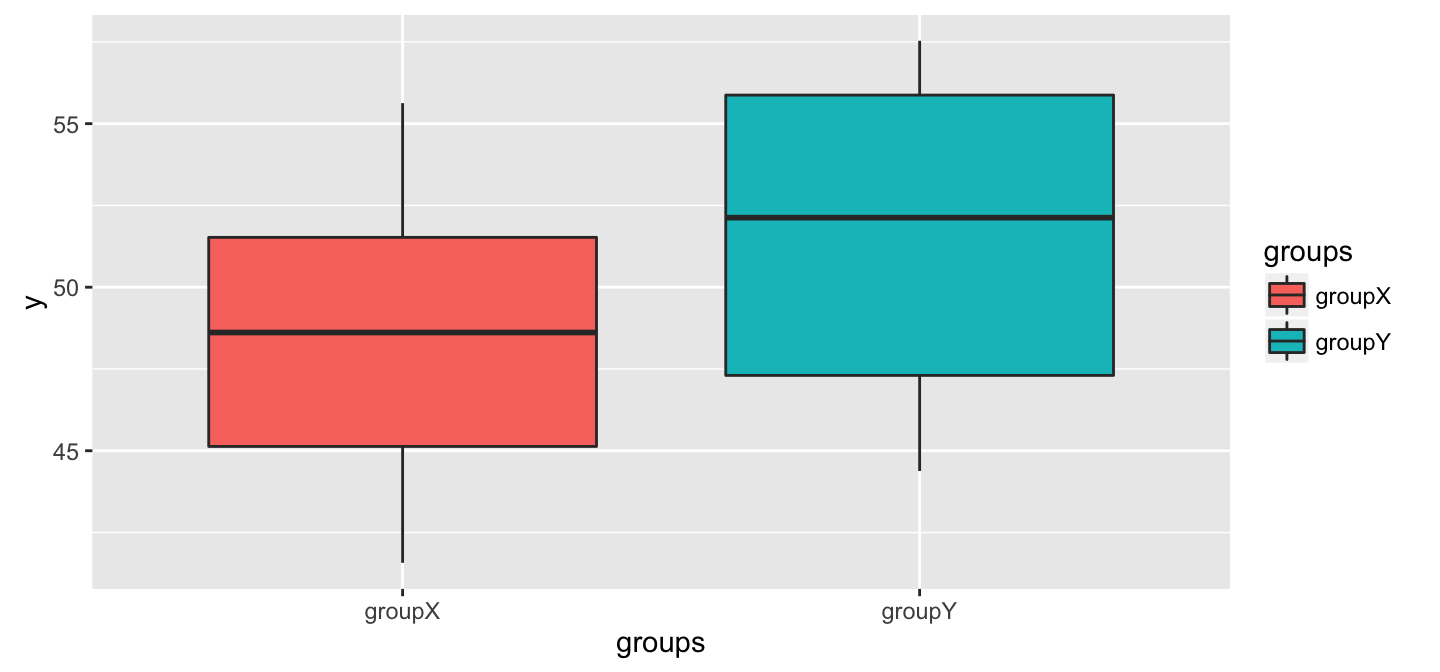## Happy Halloween## Power Analysis allows you to ask:

How big of a sample do I need to detect a given effect size with a given level of confidence.

## Steps in a power analysis

• decide on parameters for effect size, error magnitude
• decide how much power you want
• write a function to simulate data with your effect size, error magnitude, sample size
• write a for loop to increase sample size until desired power is achieved

## Type of errors - Redux

The Truth Retain $$H_0$$ Reject $$H_0$$
$$H_0$$ True Correct! Type I error ($$\alpha$$)
$$H_0$$ False Type II error ($$\beta$$) Correct!

In power analysis, $$H_0$$ is false, so any non-significant p values are Type II errors.

In a given bunch of simulations, power = $$1 - \beta$$

A common target is a power of 0.8

Remember, this is a balancing act! The more powerful, the more chance of commiting Type I error, because Type I and Type II are inversely related.

## You already wrote a function for ANOVA power analysis, in your homework!

doANOVA <- function(sampleSize=20, grandMean=50, errorSD=5, meanDiff=3, showPlot=TRUE) {
library(ggplot2)
myError <- rnorm(sampleSize, mean = 0, sd = errorSD)
groups <- rep(c("groupX", "groupY"), sampleSize/2)
y <- grandMean + c((meanDiff/2)*-1, (meanDiff/2)) + myError
if(showPlot == TRUE) print(qplot(x=groups, y=y, geom="boxplot", fill=groups))
myModel <- lm(y~groups)
return(pf(summary(myModel)$fstatistic, summary(myModel)$fstatistic,
summary(myModel)\$fstatistic,
lower.tail = FALSE))
}

## We are set up to do a power analysis

doANOVA(sampleSize=20, errorSD=5, meanDiff=3)##     value
## 0.1553212

## Calculate power for a given sample size

To figure out $$beta$$ (Type II error rate) for a given sample size, we need to run our function a bunch of times

calculatePower <- function(n){
results<-numeric(500)
for(i in 1:500){
results[i] <- doANOVA(sampleSize=n, errorSD=5, meanDiff=3, showPlot=FALSE)
}
beta <- sum(results>0.05) / length(results)
return(1 - beta)
}
calculatePower(n=20)
##  0.252
At this sample size, we aren't very consistently detecting this mean difference between groups.

## Let's figure out how large our sample size needs

We want to detect our effect at least 80% of the time

So, we can start with our sample size of 20, which we know is too low, and increment our sample size up and up until we hit a power of 0.8

## Another loop to find n with power >= 0.8

currentPower <- 0
sampleSize <- 20

while(currentPower < 0.8){
currentPower <- calculatePower(n=sampleSize)
sampleSize <- sampleSize + 4
}
currentPower
##  0.814
sampleSize
##  96